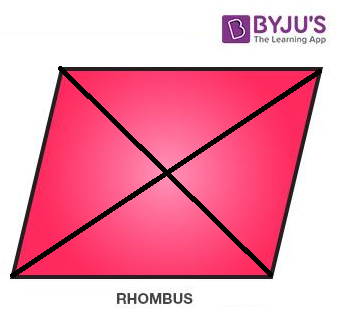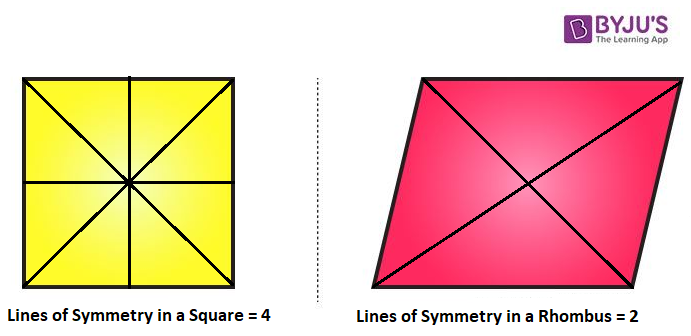Jet Set Go! All about Aeroplanes Jet Set Go! All about Aeroplanes

# Rhombus Lines of Symmetry

A rhombus has two lines of symmetry. In a rhombus, the lines of symmetry refer to the lines which divide a rhombus into two identical halves and each half is the mirror image of the other. Different objects have a different number of symmetry lines. Here, the rhombus lines of symmetry are discussed in detail.

## What are the Lines of Symmetry in Rhombus?

A rhombus has 2 lines of symmetry which cuts it into two identical parts. Both the lines of symmetry in a rhombus are from its diagonals. So, it can be said the rhombus lines of symmetry are its both diagonals.### How are Rhombus Lines of Symmetry Different from Square Lines of Symmetry?

To recall, a rhombus is a 2-dimensional geometric figure whose all sides are equal. Unlike a square, the angles of a rhombus are not 90 degrees. So, the number of lines of symmetry are different for both square and rhombus. A rhombus has only two lines of symmetry, whereas a square has 4. This can be observed from the below figure.### Rotational Symmetry of a Rhombus

A rhombus has four equal sides but of varying angles. A rhombus has two lines of symmetry (vertical and horizontal), an order of two, and the angle of rotation of 180 degrees.

## Frequently Asked Questions on Rhombus Lines of Symmetry

### What is a Rhombus?

A rhombus is a quadrilateral whose all sides are equal, but angles are different. In a rhombus, the opposite sides are parallel, and the opposite angles are equal.

### How Many Lines of Symmetry Does a Rhombus Have?

A rhombus has 2 lines of symmetry. The diagonals of a rhombus are its symmetry lines.

### Does a Rhombus Have Rotational Symmetry?

Yes, a rhombus has rotational symmetry. A rhombus has rotational symmetry of 180° (Order 2).

Test your Knowledge on Rhombus Lines of Symmetry